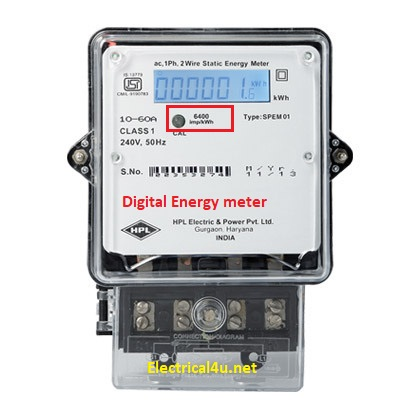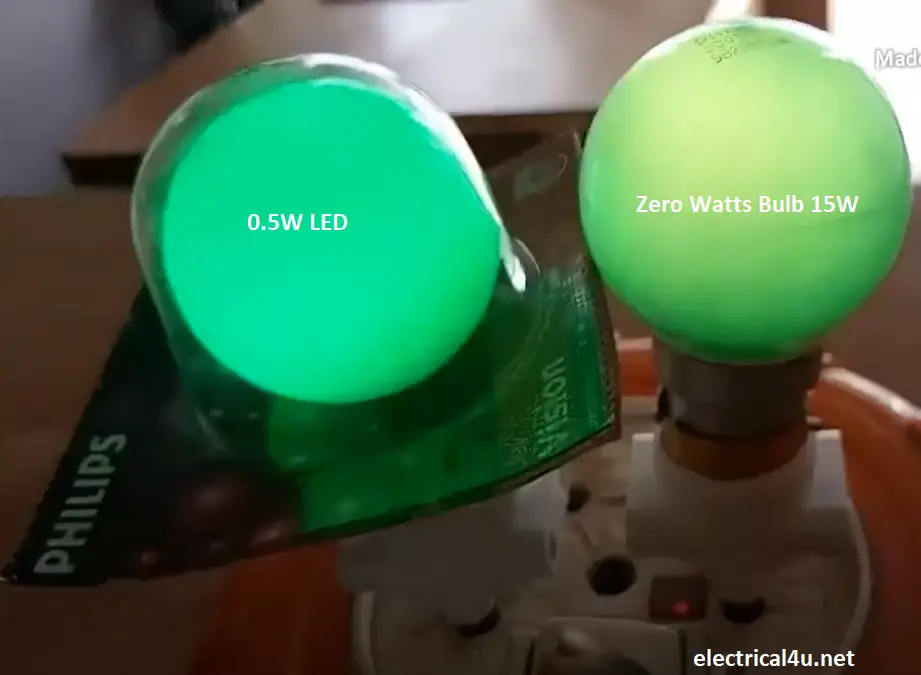# What is 0 Wattage Bulb, Power Consumption, Calculation

### 0 Watts bulb power consumption calculator

Enter the operating hours and per unit rate, then press the calculate button to get zero wattage bulb power consumption per day, per month, and per year along with the electricity bill.

 Enter Hours Used Per Day: Hr Zero Wattage Bulb: W Per Unit rate: Energy Per Day: kWh Energy Per Month: kWh Energy Per Year: kWh Cost Per Hour: Cost Per Day: Cost Per Month: Cost Per Year:

### 0-watt bulb power consumption:

As you can see in many electrical stores, if you ask for a zero wattage bulb, the shopkeeper will give you a small incandescent bulb, which is called a zero watt bulb. But actually, there is no zero watt bulbs exist in the world. This is a marketing strategy by the lamp manufacturer.

Do you know? the actual zero wattage bulb consumes 15 W for one hour.

### What is zero watts bulb?

At an earlier stage, we had used a conventional energy meter, technically electromagnetic disk type. According to our above description, the zero watts bulb wattage will be 15 watts per hour, hence per day energy consumption will be 15*24 = 360 Watt-hour, converting it into kWh, it will be 0.36kWh or 0.36 Units.

Learn More:   LED, LCD, CRT TV & Plasma TV Power Consumption, Wattage

Look at the above conventional energy meter that has a range of 600 Rev per kWh. It means to count one unit or kWh, it takes 600 revolutions.

But our zero watts bulb consumes 0.36 units per day. Hence while using such a convention meter, the net revolution will be 200 per day and the meter reading remains unchanged. Hence the people were assumed that the bulb was not really consumed any wattage.

To complete one unit, we should switch on the bulb for 3 days. Then only, the conventional energy meter counts it as one unit.

The above process was transformed into a strategy for selling zero wattage (15W) bulbs.

But later period, the digital energy meter was installed by the government; in digital energy meter, the electricity consumption will be indicated by a small impulse.Look at the above digital energy meter, which has 6400 impulses per kWh. Let us consider our case, for the consumption of 0.36kWh, the energy meter will indicate 2100 impulse in a single day.

Currently, the trend is changed and almost people well aware of it. Now, so many of us have moved to by 0.5 to 1 Watt LED bulbs instead of zero watts bulb.

However let us calculate the total electricity consumption of the zero watts bulb per hour, per day and per month, and per year.

The power consumption of zero watts bulb E(kWh-zero watt) is equal to the wattage times of the operating hours per day divided by 1000. The formula will be,

E(kWh-zero watt) = bulb wattage * hours per day.

But last LED bulbs come with the 0.5 to 1 watt in the name of zero watts bulb with the price of 75/- (1 USD).

Let us check the live demonstration for the difference between 0 watts bulb and 0.5 Watt LED Bulb.Here, the LED light intensity a little bit higher than the zero watts bulb.